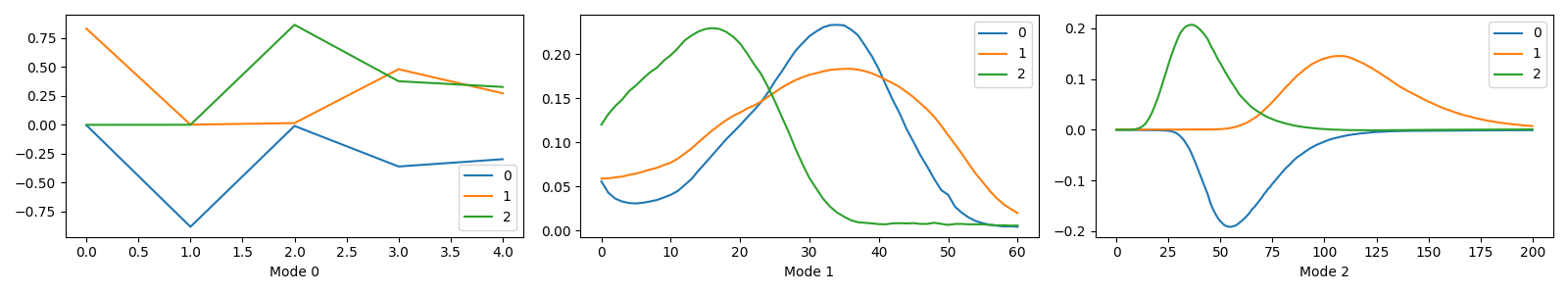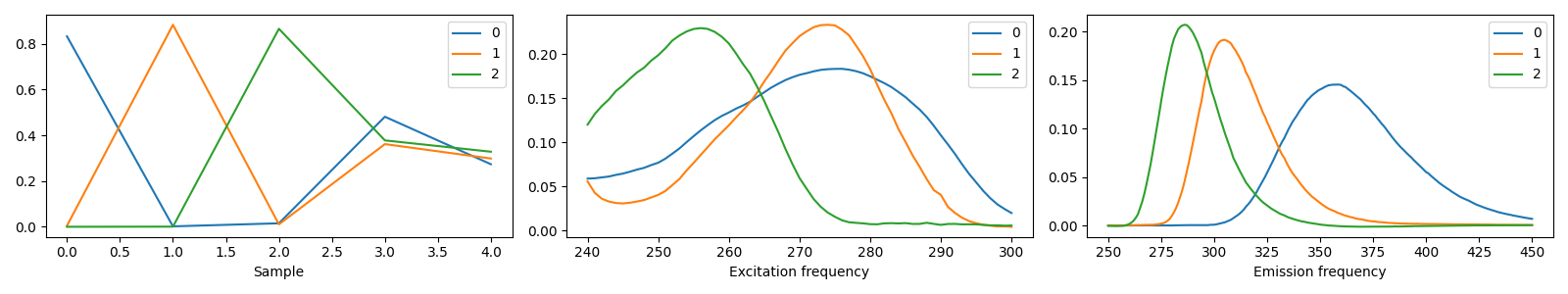# Labelled decompositions¶

TLViz uses labelled decompositions, implemented by storing the factor matrices as Pandas DataFrames. This makes it easy to make rich visualisations with the different decompositions. However, sometimes, we just want to plot the result directly from TensorLy. Luckily, this is also possible, even when we are using non-numpy backends.

## Imports and setup¶

We will set the backend to pytorch to demonstrate that TLViz also works with non-numpy backends. Note that support for non-numpy backends are experiental, so if you encounter any bugs while using non-numpy backends, please let us know by using submitting a new issue.

```import tlviz
import matplotlib.pyplot as plt
import tensorly as tl
from tensorly.decomposition import parafac

tl.set_backend("pytorch")
```

Here we load the Aminoacids dataset from [Bro97].

```aminoacids = tlviz.data.load_aminoacids()
```
```Loading Aminoacids dataset from:
Bro, R, PARAFAC: Tutorial and applications, Chemometrics and Intelligent Laboratory Systems, 1997, 38, 149-171
```

## Fitting a PARAFAC model¶

First, we fit a PARAFAC model. Normally, you should fit models using several random initialisations, but since our goal here is not data analysis, but demonstrating how TLViz visualises decompositions with and without labels, we fit only one model.

```X = tl.tensor(aminoacids.data)
cp_tensor = parafac(X, 3, init="random", random_state=0)
```

## Plotting the components¶

Next, we plot the components without postprocessing the decomposition.

```fig, ax = tlviz.visualisation.components_plot(cp_tensor)
plt.show()
```We see that the component plots have used the component vector indices for the values along the x-axis and that the x-labels get the default values, “Mode 0”, “Mode 1”, and “Mode 2”.

## Plotting the components¶

And finally, we plot the postprocessed decomposition instead.

```cp_tensor_postprocessed = tlviz.postprocessing.postprocess(cp_tensor, aminoacids)
fig, ax = tlviz.visualisation.components_plot(cp_tensor_postprocessed)
plt.show()
```We see that by postprocessing the decomposition before plotting the components, we get a more informative plot. The postprocessed decomposition has labels for the modes, which `tlviz.visualisation.components_plot()` uses for each plot’s values along the x-axis and to give each subplot a descriptive x-label. Postprocessing has also flipped the negative factor matrices (See `tlviz.postprocessing.resolve_cp_sign_indeterminacy()` for more information on how TLViz decides the sign of the component vectors).

Finally, we reset the backend to NumPy, because the other gallery examples assume that we use the NumPy backend.

```tl.set_backend("numpy")
```

Total running time of the script: ( 0 minutes 3.241 seconds)

Gallery generated by Sphinx-Gallery